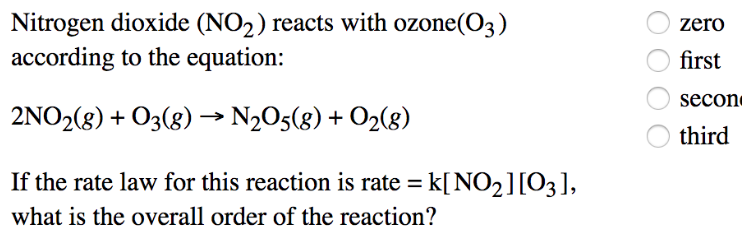# Problem: Nitrogen dioxide (NO2) reacts with ozone (O3) according to the equation: 2NO2 (g) + O3 (g) → N2O5 (g) + O2 (g) If the rate law for this reaction is rate = k[NO2] [O3], what is the overall order of the reaction? (a) zero (b) first (c) second (d) third

###### FREE Expert Solution
87% (108 ratings)###### Problem Details

Nitrogen dioxide (NO2) reacts with ozone (O3) according to the equation:

2NO(g) + O3 (g) → N2O5 (g) + O2 (g)

If the rate law for this reaction is rate = k[NO2] [O3], what is the overall order of the reaction?

(a) zero

(b) first

(c) second

(d) third